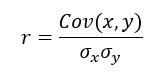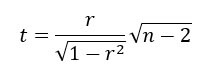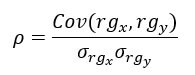# Correlation in R: Pearson & Spearman with Matrix Example

Q:
Correlation in R: Pearson & Spearman with Matrix Example

A bivariate relationship describes a relationship -or correlation- between two variables, and . In this tutorial, we discuss the concept of correlation and show how it can be used to measure the relationship between any two variables.

There are two primary methods to compute the correlation between two variables.

• Pearson: Parametric correlation
• Spearman: Non-parametric correlation
• ## Pearson Correlation

The Pearson correlation method is usually used as a primary check for the relationship between two variables.

The coefficient of correlation, , is a measure of the strength of the linear relationship between two variables and . It is computed as follow:with

The correlation ranges between -1 and 1.

• A value of near or equal to 0 implies little or no linear relationship between and .
• In contrast, the closer comes to 1 or -1, the stronger the linear relationship.

We can compute the t-test as follow and check the distribution table with a degree of freedom equals to :## Spearman Rank Correlation

A rank correlation sorts the observations by rank and computes the level of similarity between the rank. A rank correlation has the advantage of being robust to outliers and is not linked to the distribution of the data. Note that, a rank correlation is suitable for the ordinal variable.

Spearman's rank correlation, , is always between -1 and 1 with a value close to the extremity indicates strong relationship. It is computed as follow:with stated the covariances between rank and . The denominator calculates the standard deviations.

In R, we can use the cor() function. It takes three arguments, , and the method.

```cor(x, y, method)
```

Arguments:

• x: First vector
• y: Second vector
• method: The formula used to compute the correlation. Three string values:
• "pearson"
• "kendall"
• "spearman"

An optional argument can be added if the vectors contain missing value: use = "complete.obs"

We will use the BudgetUK dataset. This dataset reports the budget allocation of British households between 1980 and 1982. There are 1519 observations with ten features, among them:

• wfood: share food share spend
• wfuel: share fuel spend
• wcloth: budget share for clothing spend
• walc: share alcohol spend
• wtrans: share transport spend
• wother: share of other goods spend
• totexp: total household spend in pound
• income total net household income
• age: age of household
• children: number of children